## Example Questions

← Previous 1

### Example Question #16 : Percentage

If n% of 400 is 260, then what is 20% of n?

25

15

31

13

14

13

Explanation:

n% of 400 is 260

A number as a percentage of a second number is equal to the first number divided by the second number, times 100%.

260 / 400 * 100% = .65 * 100% = 65%. n = 65.

20% of n?

20% of 65 is equal to 65 * 20 / 100 = 65 / 5 = 13.

### Example Question #17 : Percentage

Auditions are being held for the annual school musical.  There are 18 roles available in the show.  If 75 students try out, what percentage of the students will get a role in the musical?

30%

22%

26%

28%

24%

24%

Explanation:

Percentage is #roles / # students = 18 / 75 = 0.24 = 24%

### Example Question #18 : Percentage

You are making cookies and mixing the dry ingredients: flour, white sugar and brown sugar. If the recipe calls for 3 1/4 cups of flour, 1/2 cup of white sugar and 3/4 cup of brown sugar, what percentage of the recipe is white sugar?

10%

20%

16.7%

17.5%

11.1%

11.1%

Explanation:

Total ingredients = 3 1/4 cups + 1/2 cup + 3/4 cup = 4 1/2 cups

White sugar percentage = white sugar / total = (1/2)/(9/2) = .111 = 11.1%

### Example Question #19 : Percentage

Jamie bought shoes for 30% off the regular price of \$37.99, rounded to the nearest cent. If she wanted to use exact change to pay, how much would she need in coins?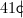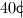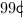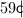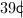Explanation: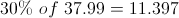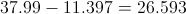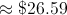### Example Question #20 : Percentage

A class has 25 students. If 60% of them are boys, how many students are girls?

18

12

10

15

10

Explanation:

If 60% of the students are boys, 40% are girls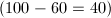. Multiply 25 by 40%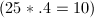. Therefore, 10 students are girls.

### Example Question #1 : How To Find The Part From The Whole With Percentage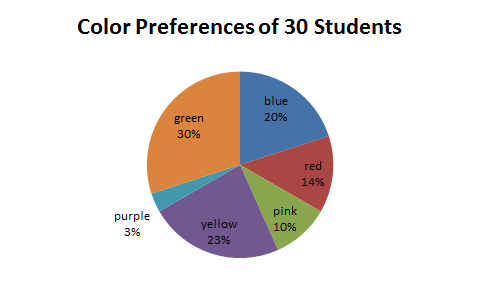The preceding graph depicts students' favorite colors. If there are 30 students in the class, how many prefer blue?

9

8

7

6

6

Explanation:

20% of the students prefer blue. We can calculate the number of students that prefer blue by multiplying the percentage by the total number of students.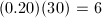### Example Question #2 : How To Find The Part From The Whole With Percentage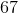is what part of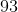?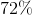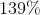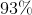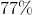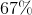Explanation:

To find how much percentis of, simply dividebyand multiply that answer by 100.

Thus,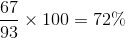### Example Question #3 : How To Find The Part From The Whole With Percentage

The Smith family consumed 35 pounds of table salt last year. As part of a healthier diet, they would like to cut their table salt consumption by 40% this year. In order to reach this goal, what is the maximum amount of salt, in pounds, that the Smith family can consume this year?

21

42

35

49

39

21

Explanation:

We need to first find 40 percent of the amount of table salt that the Smith family consumed in the previous year. In order to calculate this, we need to convert 40 percent into a decimal by moving the decimal place two spaces to the left and multiplying it by last year's salt consumption.Now, we need to subtract this amount from last year's salt consumption in order to calculate this years reduced consumption for the Smith family.### Example Question #1 : How To Find The Whole From The Part With Percentage

The average cow produces 8 gallons of milk per day. Scientists have found a vitamin supplement that can safely increase a cow’s milk production by 20%. If a dairy farm consisting of 100 cows uses the supplement, how many gallons of milk will the dairy farm now produce? (Assume each cow produces the average amount of milk per day).

1000 gallons

960 gallons

800 gallons

160 gallons

960 gallons

Explanation:

First, determine the amount of milk cows will produce with the supplement: 8 + (.2 x 8)= 9.6 gallons per day. Next, determine the amount of milk 100 cows will produce when receiving the supplement: 100 x 9.6 = 960 gallons.

### Example Question #2 : How To Find The Whole From The Part With Percentage

Florida Clementines Corp sells only the best clementines. The inspector rejected 50% of January’s harvest. Unfortunately, 20% of the inspected clementines fell off the delivery truck. If the truck delivers 200 clementines, how many were harvested in January?

500

80

285

350

500

Explanation:

We first obtain how many clementines were loaded on the delivery truck from 200 delivered clementines. Then we obtain how many clementines were inspected. 200 = (1-0.2)x gives us 250 loaded onto the delivery truck. 250 = (1-0.5)x gives us 500 harvested clementines.

← Previous 1

### All ACT Math Resources